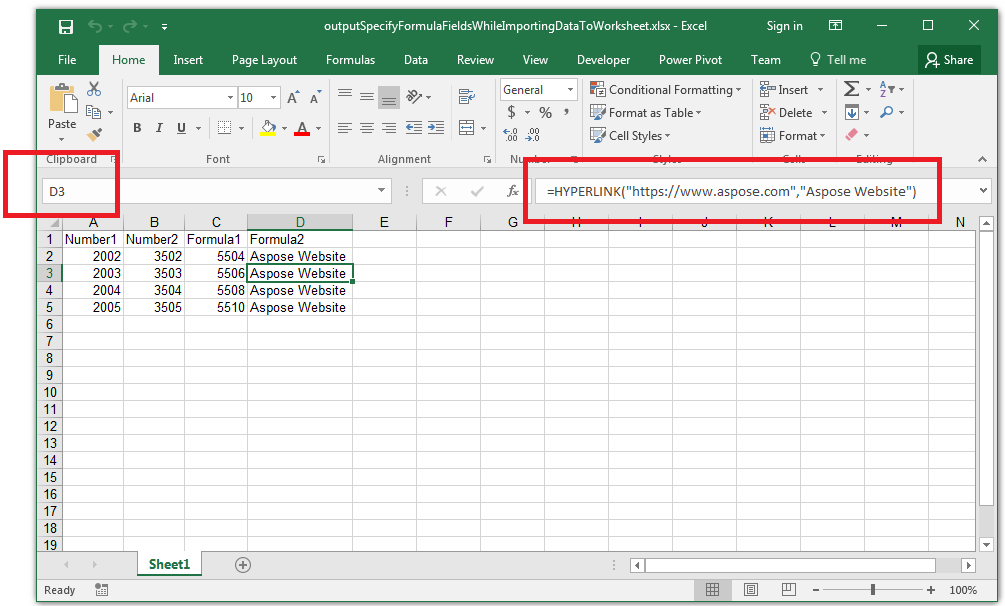# Specify Formula Fields while Importing Data to Worksheet

Contents
[ ]

## Possible Usage Scenarios

You can specify formula fields when you import data into your worksheet using the ImportTableOptions.setFormulas() method. This method takes the Boolean array where the value true means the field is a formula field. For example, if the third field is a formula field, then the third value in the array will be true.

## Specify Formula Fields while Importing Data to Worksheet

Please see the following sample code that explains how to specify the formula field while importing data to a worksheet. Please see the output Excel file generated by the code and the screenshot showing the effect of the code on the output Excel file.